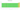# ﷼

## Iranian Rial

#### how has the value of the currency changed in the last year?## USD 1 = IRR 42,207

How much is10 US dollars worth inIranian rials?
At the current exchange rate, 10 US dollars is worth 422,075 Iranian rials
How much is50 US dollars worth inIranian rials?
At the current exchange rate, 50 US dollars is worth 2,110,375 Iranian rials
How much is100 US dollars worth inIranian rials?
At the current exchange rate, 100 US dollars is worth 4,220,749.99 Iranian rials
How much is500 US dollars worth inIranian rials?
At the current exchange rate, 500 US dollars is worth 21,103,749.96 Iranian rials
How much is2,000 US dollars worth inIranian rials?
At the current exchange rate, 2,000 US dollars is worth 84,414,999.86 Iranian rials
How much is10 US dollars worth inIranian rials?
At the current exchange rate, 10 US dollars is worth 422,075 Iranian rials
How much is50 US dollars worth inIranian rials?
At the current exchange rate, 50 US dollars is worth 2,110,375 Iranian rials
How much is100 US dollars worth inIranian rials?
At the current exchange rate, 100 US dollars is worth 4,220,749.99 Iranian rials
How much is500 US dollars worth inIranian rials?
At the current exchange rate, 500 US dollars is worth 21,103,749.96 Iranian rials
How much is2,000 US dollars worth inIranian rials?
At the current exchange rate, 2,000 US dollars is worth 84,414,999.86 Iranian rials